GATE  >  Test: Structural Analysis- 1

# Test: Structural Analysis- 1

Test Description

## 15 Questions MCQ Test GATE Civil Engineering (CE) 2023 Mock Test Series | Test: Structural Analysis- 1

Test: Structural Analysis- 1 for GATE 2023 is part of GATE Civil Engineering (CE) 2023 Mock Test Series preparation. The Test: Structural Analysis- 1 questions and answers have been prepared according to the GATE exam syllabus.The Test: Structural Analysis- 1 MCQs are made for GATE 2023 Exam. Find important definitions, questions, notes, meanings, examples, exercises, MCQs and online tests for Test: Structural Analysis- 1 below.
Solutions of Test: Structural Analysis- 1 questions in English are available as part of our GATE Civil Engineering (CE) 2023 Mock Test Series for GATE & Test: Structural Analysis- 1 solutions in Hindi for GATE Civil Engineering (CE) 2023 Mock Test Series course. Download more important topics, notes, lectures and mock test series for GATE Exam by signing up for free. Attempt Test: Structural Analysis- 1 | 15 questions in 45 minutes | Mock test for GATE preparation | Free important questions MCQ to study GATE Civil Engineering (CE) 2023 Mock Test Series for GATE Exam | Download free PDF with solutions
 1 Crore+ students have signed up on EduRev. Have you?
Test: Structural Analysis- 1 - Question 1

### A beam carries a uniformly distributed load throughout its length. In which of the following configurations will the strain energy be maximum?

Detailed Solution for Test: Structural Analysis- 1 - Question 1

Strain Energy in beams is basically equals to integration of square of Bending Moment over the length divided by EI.
For a given beam, higher the static indeterminacy lower the bending moment is developed in the cross-section, thus, resulting in lower strain energy.
Moreover, if the indeterminacy is same then, lower the number of supports, higher will be bending moment generated thus, leading to higher strain energy.
From above statements, we can conclude that cantilever has maximum strain energy.

Test: Structural Analysis- 1 - Question 2

### The force in member AB of the truss shown below is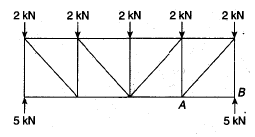Detailed Solution for Test: Structural Analysis- 1 - Question 2

If we consider the joint equilibrium at B, the force in member AB will be zero.

Test: Structural Analysis- 1 - Question 3

### The force in member BD of the truss shown below is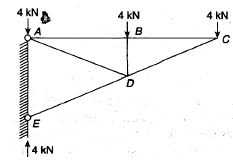Detailed Solution for Test: Structural Analysis- 1 - Question 3

If we consider the joint equilibrium at b, the force in member BD will be 4 kN compressive.

Test: Structural Analysis- 1 - Question 4

Which one of the following structures is statically determinate and stable?

Detailed Solution for Test: Structural Analysis- 1 - Question 4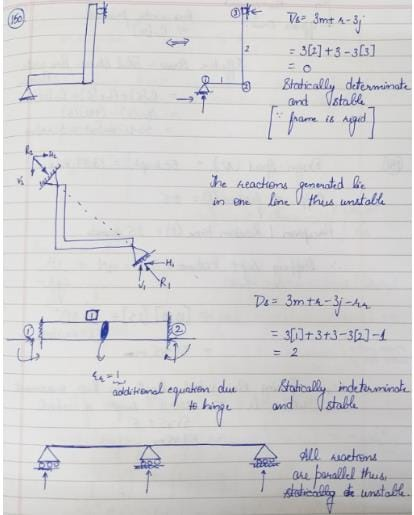Test: Structural Analysis- 1 - Question 5

For a two-hinged arch, if one of the supports settles down vertically, then the horizontal thrust

Test: Structural Analysis- 1 - Question 6

The number of independent equations to be satisfied for static equilibrium in a space structure is

Detailed Solution for Test: Structural Analysis- 1 - Question 6

Summation of forces and moments in all three directions need to be zero
Fx=0; Fy=0; Fz=0; Mx=0; My=0; Mz=0

Test: Structural Analysis- 1 - Question 7

A propped cantilever is acted upon by a moment M0 at the propped end. What is the prop action?

Detailed Solution for Test: Structural Analysis- 1 - Question 7

A structure is statically determinate when the external reactions can be determined from force-equilibrium equations.
A structure is stable when the whole or part of the structure is prevented from large displacements on account of loading.
In (a), the structure is stable and there are three reaction components that can be determined from two force equilibrium conditions and one moment equilibrium condition.
But in case of (b), the structure is stable but statically indeterminate to the second degree and in case of (c), the structure has both reaction components coinciding with each other, so the moment equilibrium conditions will never be satisfied and the structure will not be under equilibrium.
In (d), the structure is simply unstable.

Test: Structural Analysis- 1 - Question 8

The ratio of the maximum deflection between a simply supported beam carrying a point load at its midspan and the same beam carrying the seem load as total load of uniformly distributed load will be

Detailed Solution for Test: Structural Analysis- 1 - Question 8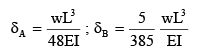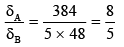Test: Structural Analysis- 1 - Question 9

For the beam shown in the below figure, to have the maximum positive bending moment equal to negative bending moment, the value of L1 has to be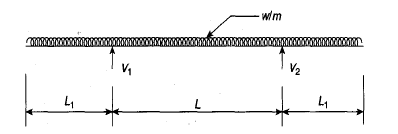Detailed Solution for Test: Structural Analysis- 1 - Question 9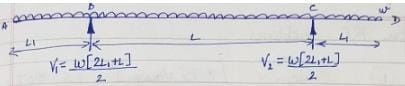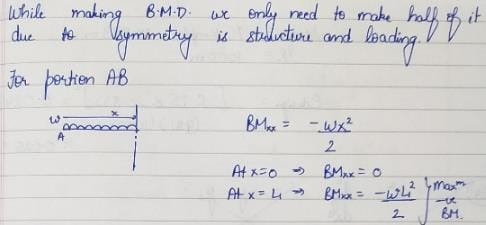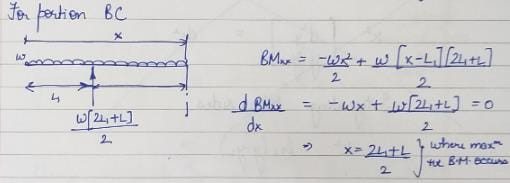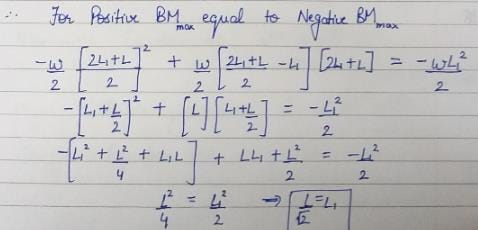Test: Structural Analysis- 1 - Question 10

A propped cantilever of uniform flexural rigidity is loaded as shown in the given figure. The bending moment at fixed end is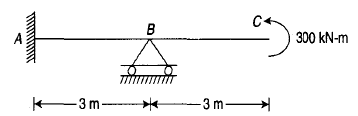Detailed Solution for Test: Structural Analysis- 1 - Question 10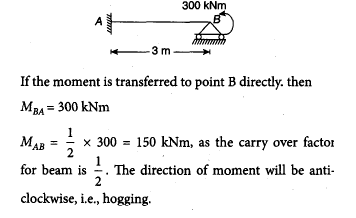Test: Structural Analysis- 1 - Question 11

The strain energy due to bending in the cantilever beam shown in the figure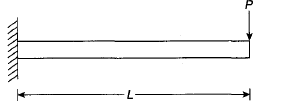Detailed Solution for Test: Structural Analysis- 1 - Question 11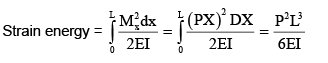Test: Structural Analysis- 1 - Question 12

In the portal frame shown in the given figure, the ratio of sway moments in column AB and CD will be equal to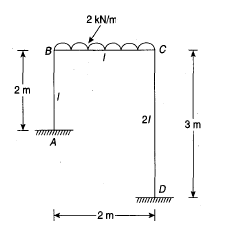Detailed Solution for Test: Structural Analysis- 1 - Question 12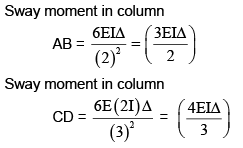Therefore, the ratio of sway moments in columns AB and CD will be equal to 9/8.

Test: Structural Analysis- 1 - Question 13

The total degree of static indeterminacy of the plane frame shown in the given figure is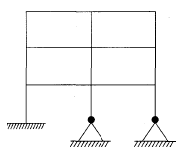Detailed Solution for Test: Structural Analysis- 1 - Question 13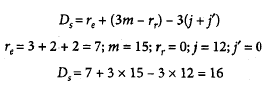Test: Structural Analysis- 1 - Question 14

The displacement coordinates for a beam are shown in the figure given below. Here, the stiffness matrix will be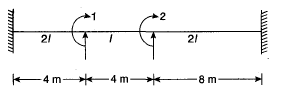Detailed Solution for Test: Structural Analysis- 1 - Question 14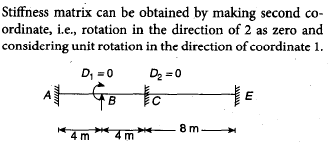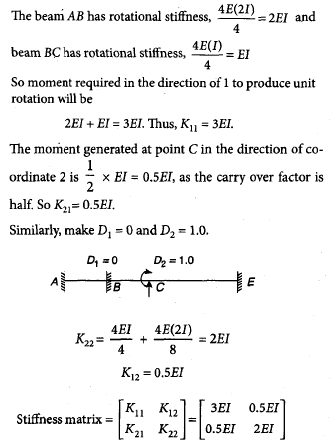Test: Structural Analysis- 1 - Question 15

The flexibility matrix for the beam shown below is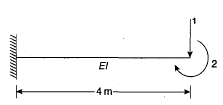Detailed Solution for Test: Structural Analysis- 1 - Question 15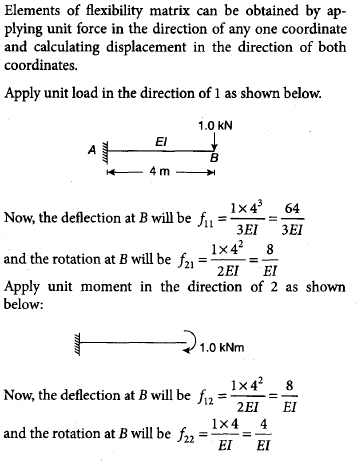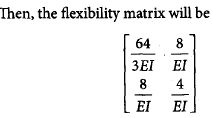## GATE Civil Engineering (CE) 2023 Mock Test Series

26 docs|292 tests
 Use Code STAYHOME200 and get INR 200 additional OFF Use Coupon Code
Information about Test: Structural Analysis- 1 Page
In this test you can find the Exam questions for Test: Structural Analysis- 1 solved & explained in the simplest way possible. Besides giving Questions and answers for Test: Structural Analysis- 1, EduRev gives you an ample number of Online tests for practice

## GATE Civil Engineering (CE) 2023 Mock Test Series

26 docs|292 tests